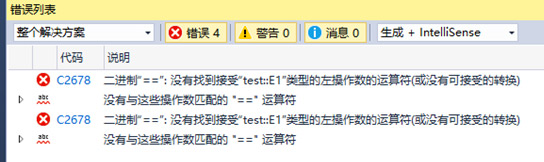# C与C++的枚举的不同[C++培训]

C语言和C++语言都提供了枚举类型，两者是有一定区别。我们接下来通过代码进行演示:

1. C语言中 enum

void test()

{

enum   Week { Mon, Tue, Wed, Thi, Fri, Sat, Sun };

enum   Other { One, Two, Three };

enum   Week week = Mon;

//   1. 允许非枚举值赋值给枚举类型, 允许其他枚举类型的值赋值给当前枚举类型

week   = 100;  //ok

week   = One;  //ok

// 2. 枚举值具有外层作用域，容易造成名字冲突

int One = 100;  //error

//   3. 不同类型的枚举值可以直接比较

if   (week == One)

{

printf("equal\n");

}

}2、C++中 enum

void test()

{

enum   Week { Mon, Tue, Wed, Thi, Fri, Sat, Sun };

enum   Other { One, Two, Three };

enum   Week week = Mon;

// 1. C++ 只能允许赋值枚举值

// week = 100;  //error

// week = One;    //error

//   2. 枚举元素会暴露在外部作用域，不同的两个枚举类型，若含有相同枚举元素，则会冲突

enum   OtherWeek { Mon };

//   3. C++ 只允许同枚举类型值之间比较

//   enum E1 { A, B };  //error

//   enum E2 { C, D };  //error

//   cout << (E1::B == E2::D ? "相等" : "不相等") << endl;  //error

}3. C++中的 enum class

void test()

{

enum   class E1 { A = 1, B = 2 };

enum   class E2 { A = 1, C = 2 };

//   1. 强枚举类型不会将枚举元素暴露在外部作用域

cout   << (int)(E1::A) << endl;

int   A = 100;

//   2. 不相关的两个枚举类型不能直接比较，编译报错

cout   << (E1::B == E2::C ? "相等" : "不相等") << endl; //error

cout   << (E1::B == 2 ? "相等" : "不相等") << endl; //error

}4. 总结

1. C 枚举类型支持不同类型枚举值之间赋值、以及数字赋值、比较，并且具有外层作用域。

2. C++ 中枚举不允许不同类型的值给枚举类型变量赋值，但仍然支持不同类型之间枚举进行比较，枚举符号常量具有挖外作用域。

3. C++ 强枚举类型不允许不同类型之间的赋值、比较，枚举常量值并不具有外层作用域。推荐了解黑马程序员C++培训课程。

C++与C如何区分？

c语言编程入门视频教程下载

JavaEE